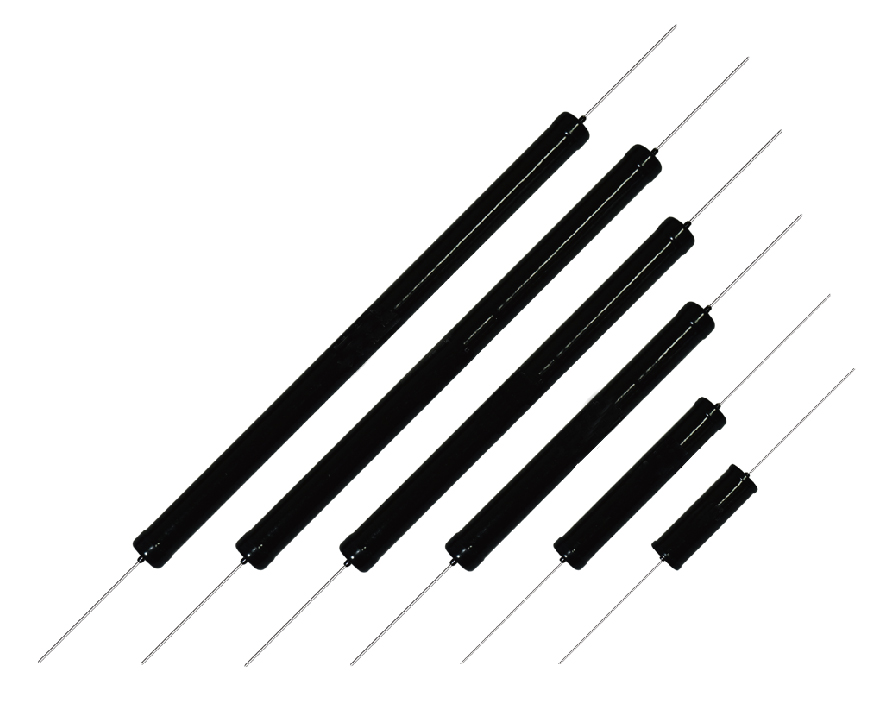Tel:400-803-9333
• Welcome to MicrohmPosition：Home » Technical Articles

Resistance Of A Resistor

Writer：Microhm Page View：Date：2019-07-25
The function of a resistor is to oppose the electric current through it. This is called electrical resistance, and is measured in the unit ohm. The resistance can be calculated with Ohms law, when the current is known and the voltage drop is measured by Ohm's Law R=V/I. Recently more customers request for TΩ resistors or GΩ ( gigaohm ) resistors for measurement and control equipment and other applications. Microhm offer thick film resistor NRB series and NGP type.The concept of current, voltage and resistance can be explained by a hydraulic analogy. A flow of water through a pipe is restricted by a constriction. This causes a pressure drop after the constriction. The flow of water is equivalent to electric current. The pressure drop is equal to the voltage drop. The constriction is equivalent to the resistor, and has a certain resistance. The resistance is proportional to the voltage or pressure drop for a given current.

In the hydraulic example, the resistance can be increased by for example reducing the diameter of the constriction. For a resistor or wire, the resistance is in general dependent on the material and the geometrical shape. The influence of the geometrical shape, can easily be explained by using the hydraulic example. A long and narrow tube will have a higher resistance than a short and wide tube.The resistance of a resistor is dependent on its material and shape. Some materials have a higher resistivity, causing a higher value. The value is often printed on the resistor with a number or in the form of a color code.

Resistance is dependent on the geometrical shape and material. The resistance of a resistor is either printed on the resistor body or marked with a color code. The combination of colors indicated the value and tolerance of a resistor.

Keywords：